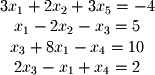We now come to the first major application of the basic techniques of linear algebra: solving systems of linear equations. In elementary algebra, these systems were commonly called simultaneous equations. For example, given the following simultaneous equations, what are the solutions for x, y, and z? (This system will be used as an example in many of the subsequent subsections of this chapter)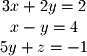The two common methods of solving this system are by substitution (solving, say, the second equation for x and substituting this value into the first and third to solve for x and z) or by elimination (multiplying the second by 2 and adding it to the first and then multiplying the second by 5 and adding it to the third). Either method yields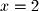,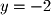, and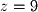.

Although substitution or elimination is tractable for a few equations with a few unknowns, large sets of unknowns and equations require more computationally efficient methods. All of these methods are based on writing the system in several matrices: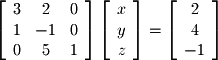This form is called the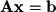form of a system where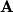is called the coefficient matrix,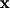is the unknowns or solution vector, and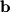is the constant vector. The general form of such a system with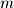equations and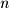unknowns is given by the following: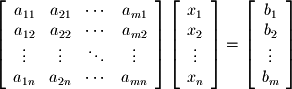Note that the number of rows always equals the number of equations so the vertical height of the coefficient matrixalways equals the length of the constant vector(every equation has to have a corresponding constant even if that constant is 0). Likewise, the horizontal length of the matrix (number of columns) equals the length of the unknowns vector.

It is common to write the system Ax=b in augmented matrix form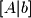: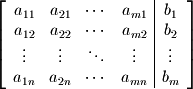The next few subsections discuss some of the basic techniques for solving systems in this form. More advanced techniques are saved for later chapters.
Excercise 5-1.
Write the following system of equations in augmented form: